Next: About this document ... Up: lab_template Previous: lab_template

Subsections

# MA 1024: Surfaces

## Purpose

The purpose of this lab is to introduce you to some of the Maple commands that can be used to plot surfaces in three dimensions.

## Getting Started

To assist you, there is a worksheet associated with this lab that contains examples to get you aquainted with the lab. You can copy that worksheet to your home directory with the following command, which must be run in a terminal window for example, not in Maple.

cp /math/calclab/MA1024/Surf_start.mws My_Documents


You can copy the worksheet now, but you should read through the lab before you load it into Maple. Once you have read to the exercises, start up Maple, load the worksheet Surf_start.mws, and go through it carefully. Then you can start working on the exercises.

## Background

The graph of a function of a single real variable is a set of points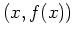in the plane. Typically, the graph of such a function is a curve. For functions of two variables in Cartesian coordinates, the graph is a set of points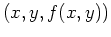in three-dimensional space. For this reason, visualizing functions of two variables is usually more difficult.

One of the most valuable services provided by computer software such as Maple is that it allows us to produce intricate graphs with a minimum of effort on our part. This becomes especially apparent when it comes to functions of two variables, because there are many more computations required to produce one graph, yet Maple performs all these computations with only a little guidance from the user.

The simplest way of describing a surface in Cartesian coordinates is as the graph of a functionover a domain, e.g. a set of points in the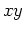plane. The domain can have any shape, but a rectangular one is the easiest to deal with.

Another common, but more difficult way of describing a surface is as the graph of an equation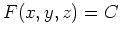, where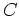is a constant. In this case, we say the surface is defined implicitly. A third way of representing a surfaceis through the use of level curves. The idea is that a plane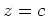intersects the surface in a curve. The projection of this curve on theplane is called a level curve. A collection of such curves for different values of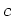is a representation of the surface called a contour plot.

Similar to the idea of level curves is to look at cross sections of the surface to see what two-dimensional shape is traced, not only in theplane by letting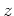be constant, but also in the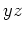plane by holdingconstant and the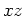plane by holdingconstant.

## Exercises

1. For the given equations below, plot two dimensional level curves parallel to theplane and then plot two dimensional cross sections in theplane and theplane. Identify the type or shape of the quadric surface, ie. a sphere, cylinder, cone, elliptic cone, paraboloid, elliptic parabaloid, ellipsoid, hyperboloid of one sheet, hyperboloid of two sheets, elliptic hyperboloid of one or two sheets, or a hyperbolic parabaloid (saddle). Once you have determined the shape of the surface, supply a three dimensional plot to support your conclusion.
a)b)c)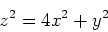d)2. Create a contour plot for the function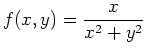for thevalues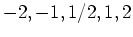using two different methods; first using cross sections and then using Maple's contourplot command.

3. Consider the following function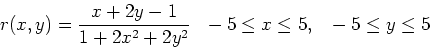which hypothetically represents the deviation, in inches, of last year's rainfall from the average annual rainfall in a certain area. Graph the surface corresponding to the function. Use axes that are boxed. Based on the graph, approximately what are the maximum and minimum values of the rainfall deviation?Next: About this document ... Up: lab_template Previous: lab_template
Dina Solitro
2007-03-20